# Colleges with the highest SAT scores in Iowa

Top 10 colleges in Iowa with the highest SAT scores
Looking for the colleges with the highest SAT scores in Iowa? Well you're in luck! We've compiled a national college database and have created a list of the top 10 universities with the highest SAT scores in Iowa. These are the schools whose applicants had the highest average SAT scores in Iowa. And, since these tests are meant to determine academic prowess, they are arguably the schools with the most academically proficient students. You could even say these are the best colleges in Iowa. We also include each college's ACT scores and acceptance rate so that you can see where you would have the easiest or hardest time getting in. Read on to find out more.

## Grinnell College SAT scores

The average SAT score for Grinnell College is 1460.The average SAT score of 1460 breaks down into:

• SAT math: 750

The average ACT score for Grinnell College is 32 and their acceptance rate is 24.4%.

## Drake University SAT scores

The average SAT score for Drake University is 1248.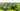The average SAT score of 1248 breaks down into:

• SAT math: 620

The average ACT score for Drake University is 27 and their acceptance rate is 67.7%.

## Univeristy of Iowa SAT scores

The average SAT score for Univeristy of Iowa is 1235.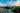The average SAT score of 1235 breaks down into:

• SAT math: 625

The average ACT score for Univeristy of Iowa is 26 and their acceptance rate is 82.6%.

## Dordt College SAT scores

The average SAT score for Dordt College is 1230.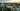The average SAT score of 1230 breaks down into:

• SAT math: 630

The average ACT score for Dordt College is 25 and their acceptance rate is 75.4%.

## Iowa State University SAT scores

The average SAT score for Iowa State University is 1220.The average SAT score of 1220 breaks down into:

• SAT math: 625

The average ACT score for Iowa State University is 25 and their acceptance rate is 92.1%.

## Cornell College SAT scores

The average SAT score for Cornell College is 1210.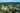The average SAT score of 1210 breaks down into:

• SAT math: 600

The average ACT score for Cornell College is 27 and their acceptance rate is 61%.

## Coe College SAT scores

The average SAT score for Coe College is 1200.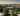The average SAT score of 1200 breaks down into:

• SAT math: 610

The average ACT score for Coe College is 25 and their acceptance rate is 56.2%.

## Simpson College SAT scores

The average SAT score for Simpson College is 1180.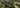The average SAT score of 1180 breaks down into:

• SAT math: 590

The average ACT score for Simpson College is 24 and their acceptance rate is 82.5%.

## Luther College SAT scores

The average SAT score for Luther College is 1165.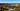The average SAT score of 1165 breaks down into:

• SAT math: 590

The average ACT score for Luther College is 26 and their acceptance rate is 65.1%.

## Saint Ambrose University SAT scores

The average SAT score for Saint Ambrose University is 1140.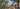The average SAT score of 1140 breaks down into:

• SAT math: 570